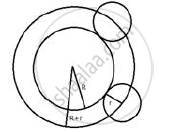# Describe: the Locus of the Centres of a Given Circle Which Rolls Around the Outside of a Second Circle and is Always Touching It. - Mathematics

Sum

Describe:

The locus of the centres of a given circle which rolls around the outside of a second circle and is always touching it.

#### Solution

The locus is the circumference of the circle concentric with the second circle whose radius is equal to the sum of the radii of the two given circles.Concept: Introduction of Loci
Is there an error in this question or solution?

#### APPEARS IN

Selina Concise Maths Class 10 ICSE
Chapter 16 Loci (Locus and Its Constructions)
Exercise 16 (B) | Q 15.5 | Page 241